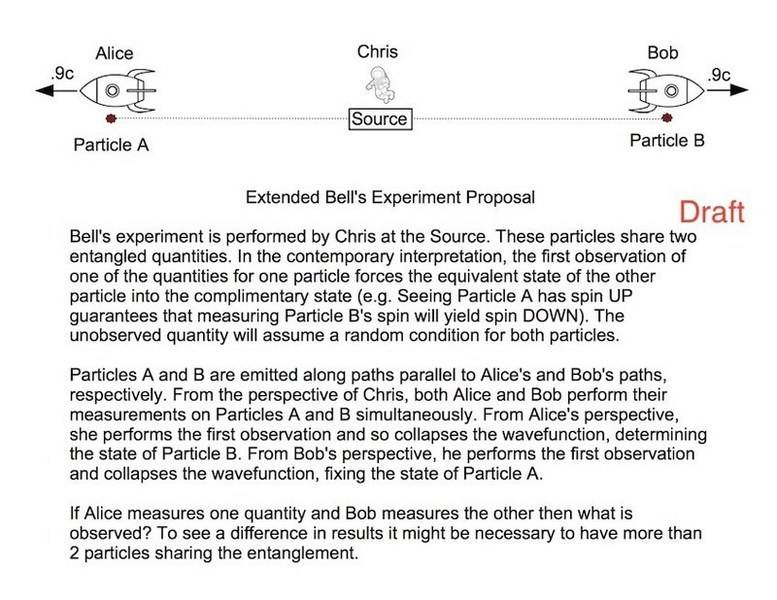# Order of observations in Bell experiments

• B
• Android Neox

#### Android Neox

I've been trying to find information on how Bell's experiment works on the macroscopic scale. I think that the scale of current measurements is meters, for the most part. I've been curious about how Bell's Inequality is supposed to work, Relativistically. Haven't found quite what I'm looking for. Non-simultaneity means that different frames can see different observers collapse the wavefunction. What happens if two observers see themselves as making the first observation but of different entangled quantities? Attached, below, is what I've been messing with. If someone could direct me to existing work on Bell with different reference frames, I'd be grateful.

It has been explained to me that you don't do science here but I've not been able to find anything on this topic and thought someone here might be able to direct me to something.Last edited by a moderator:

. I've been curious about how Bell's Inequality is supposed to work, Relativistically. Haven't found quite what I'm looking for. Non-simultaneity means that different frames can see different observers collapse the wavefunction. What happens if two observers see themselves as making the first observation but of different entangled quantities?

Alice and Bob both make measurements and get results. Depending on their relative speeds, some observers will say that Alice's measurement came first, others will say that Bob's measurement came first, and observers at rest relative to Chris will say that both measurements happened at the same time. At some later time - all observers will agree that this happens after both of the measurements - we compare Alice's and Bob's notes to see how their measurements are correlated. (To actually prove the correlation and that we're dealing with entangled pairs we'd need to repeat this process many times with many pairs. If Bob and Alice measure on the same axis and get oppoiste results once, that's just happenstance, happens half the time even if the particles are not entangled. But if they try hundreds of pairs, and it turns out that way every single time... then we know that something is going on).

It makes no difference which observation we say came first. Let's say, for definiteness that Bob measured spin-up and Alice measured spin-down. We could say that Bob went first and his "up" measurement collapsed the wave function to "Bob-up, Alice-down" so that Alice was fated to measure "down"; or we could say that Alice went first and her "down" measurement collapsed the wave function to "Bob-up, Alice-down" so that Bob was fated to measure "up". Both descriptions lead to exactly the same physical result: Bob measures spin-up, Alice measures spin-down. Thus, relativity of simultaneity is not a problem here - the outcome is the same whether we describe it using a frame in which Alice's measurement was first, Bob's measurement was first, or both happened at the same time.
If someone could direct me to existing work on Bell with different reference frames...
This question suggests that you are the victim of a (very common) misconception about reference frames. Events don't happen in reference frames - they happen and then (optionally) we choose some reference frame and use it to assign x, y, z, and t coordinates to them. Thus everything is always in every imaginable reference frame already, and that includes the labs where in which Bell experiments are being done.
(If this is not clear to you, you may find some helpful threads over in the Relativity subforum).

There is a strange sort of symmetry here, that I can illustrate if we take an entangled photon pair fired at polarization filters instead of an electron pair: Suppose filter A is at angle α and filter B at angle β. If we assume that photon A arrives first at filter A, it collapses to polarization direction α. Now, photon B has probability amplitude cos(β-α) to pass filter B. Conversely, if photon B arrives first at filter B, it collapses to polarization direction β, leaving A a probability amplitude of cos(α-β) of passing filter A. When the information of the two is combined we obtain probability cos(β-α)*cos(α-β) of correlation. This is symmetrical. The same holds for measuring entangled particle spins at some angle.

Last edited: